Skip to main content Accessibility help
Home
Hostname: page-component-747cfc64b6-4xs5l Total loading time: 0.229 Render date: 2021-06-15T20:51:19.080Z Has data issue: true Feature Flags: { "shouldUseShareProductTool": true, "shouldUseHypothesis": true, "isUnsiloEnabled": true, "metricsAbstractViews": false, "figures": true, "newCiteModal": false, "newCitedByModal": true, "newEcommerce": true }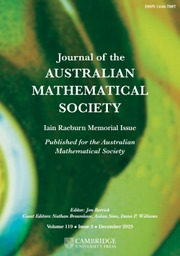Journal of the Australian Mathematical Society

# MÖBIUS INVARIANT FUNCTION SPACES AND DIRICHLET SPACES WITH SUPERHARMONIC WEIGHTS

Published online by Cambridge University Press:  12 July 2018

Corresponding
E-mail address:

## Abstract

Let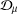${\mathcal{D}}_{\unicode[STIX]{x1D707}}$ be Dirichlet spaces with superharmonic weights induced by positive Borel measures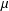$\unicode[STIX]{x1D707}$ on the open unit disk. Denote by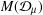$M({\mathcal{D}}_{\unicode[STIX]{x1D707}})$ Möbius invariant function spaces generated by${\mathcal{D}}_{\unicode[STIX]{x1D707}}$. In this paper, we investigate the relation among${\mathcal{D}}_{\unicode[STIX]{x1D707}}$,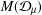$M({\mathcal{D}}_{\unicode[STIX]{x1D707}})$ and some Möbius invariant function spaces, such as the space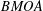$BMOA$ of analytic functions on the open unit disk with boundary values of bounded mean oscillation and the Dirichlet space. Applying the relation between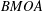$BMOA$ and$M({\mathcal{D}}_{\unicode[STIX]{x1D707}})$, under the assumption that the weight function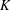$K$ is concave, we characterize the function$K$ such that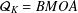${\mathcal{Q}}_{K}=BMOA$. We also describe inner functions in$M({\mathcal{D}}_{\unicode[STIX]{x1D707}})$ spaces.

## MSC classification

Type
Research Article
Information
Copyright
© 2018 Australian Mathematical Publishing Association Inc.

## Access options

Get access to the full version of this content by using one of the access options below.

## Footnotes

G. Bao and H. Wulan were supported by the NNSF of China (No. 11720101003). G. Bao was also supported by the STU Scientific Research Foundation for Talents (No. NTF17020).

Current address: Department of Mathematics and Statistics, Texas Tech University, Lubbock, Texas 79409, USA stamatis.pouliasis@ttu.edu

## References

Aleman, A., ‘Hilbert spaces of analytic functions between the Hardy and the Dirichlet space’, Proc. Amer. Math. Soc. 115 (1992), 97104.CrossRefGoogle Scholar
Aleman, A., ‘The multiplication operator on Hilbert spaces of analytic functions’, Habilitation, FernUniversität in Hagen, 1993.Google Scholar
Aleman, A. and Simbotin, A., ‘Estimates in Möbius invariant spaces of analytic functions’, Complex Var. Theory Appl. 49 (2004), 487510.Google Scholar
Arazy, J., Fisher, S. and Peetre, J., ‘Möbius invariant function spaces’, J. reine angew. Math. 363 (1985), 110145.Google Scholar
Armitage, D. H. and Gardiner, S. J., Classical Potential Theory, Springer Monographs in Mathematics (Springer, London, 2001).CrossRefGoogle Scholar
Aulaskari, R. and Lappan, P., ‘Criteria for an analytic function to be Bloch and a harmonic or meromorphic function to be normal’, in: Complex Analysis and its Applications, Pitman Research Notes in Mathematics, 305 (Longman Scientific and Technical, Harlow, 1994), 136146.Google Scholar
Aulaskari, R., Xiao, J. and Zhao, R., ‘On subspaces and subsets of BMOA and UBC ’, Analysis 15 (1995), 101121.CrossRefGoogle Scholar
Axler, S., ‘The Bergman space, the Bloch space, and commutators of multiplication operators’, Duke Math. J. 53 (1986), 315332.CrossRefGoogle Scholar
Baernstein, A., Analytic Functions of Bounded Mean Oscillation, Aspects of Contemporary Complex Analysis (Academic Press, London–New York, 1980), 336.Google Scholar
Bao, G., Göğüş, N. G. and Pouliasis, S., ‘On Dirichlet spaces with a class of superharmonic weights’, Canad. J. Math. doi:10.4153/CJM-2017-005-1.Google Scholar
Bao, G., Göğüş, N. G. and Pouliasis, S., ‘𝓠 p spaces and Dirichlet type spaces’, Canad. Math. Bull. 60 (2017), 690704.CrossRefGoogle Scholar
Bao, G., Lou, Z., Qian, R. and Wulan, H., ‘Improving multipliers and zero sets in 𝓠 K spaces’, Collect. Math. 66 (2015), 453468.CrossRefGoogle Scholar
Carleson, L., ‘An interpolation problem for bounded analytic functions’, Amer. J. Math. 80 (1958), 921930.CrossRefGoogle Scholar
Carleson, L., ‘Interpolation by bounded analytic functions and the corona problem’, Ann. of Math. 76 (1962), 547559.CrossRefGoogle Scholar
Duren, P. L., Theory of H p Spaces (Academic Press, New York–London, 1970). Reprinted with supplement by Dover Publications, Mineola, New York, 2000.Google Scholar
Duren, P. L. and Schuster, A., ‘Finite unions of interpolation sequences’, Proc. Amer. Math. Soc. 130 (2002), 26092615.CrossRefGoogle Scholar
El-Fallah, O., Kellay, K., Klaja, H., Mashreghi, J. and Ransford, T., ‘Dirichlet spaces with superharmonic weights and de Branges–Rovnyak spaces’, Complex Anal. Oper. Theory 10 (2016), 97107.CrossRefGoogle Scholar
Essén, M. and Wulan, H., ‘On analytic and meromorphic functions and spaces of 𝓠 K -type’, Illinois J. Math. 46 (2002), 12331258.Google Scholar
Essén, M., Wulan, H. and Xiao, J., ‘Several function-theoretic characterizations of Möbius invariant 𝓠 K spaces’, J. Funct. Anal. 230 (2006), 78115.CrossRefGoogle Scholar
Essén, M. and Xiao, J., ‘Some results on 𝓠 p spaces, 0 < p < 1’, J. reine angew. Math. 485 (1997), 173195.Google Scholar
Garnett, J. B., Bounded Analytic Functions. Revised 1st edn, Graduate Texts in Mathematics, 236 (Springer, 2007).Google Scholar
Girela, D., ‘Analytic functions of bounded mean oscillation’, in: Complex Function Spaces, Mekrijärvi, 1999, Department of Mathematics Report Series, 4 (ed. Aulaskari, R.) (University of Joensuu, Joensuu, 2001), 61170.Google Scholar
Gorkin, P. and Mortini, R., ‘Two new characterizations of Carleson–Newman Blaschke products’, Israel J. Math. 177 (2010), 267284.CrossRefGoogle Scholar
McDonald, G. and Sundberg, C., ‘Toeplitz operators on the disc’, Indiana Univ. J. Math. 28 (1979), 595611.CrossRefGoogle Scholar
Pau, J. and Peláez, J., ‘On the zeros of functions in Dirichlet-type spaces’, Trans. Amer. Math. Soc. 363 (2011), 19812002.CrossRefGoogle Scholar
Richter, S., ‘A representation theorem for cyclic analytic two-isometries’, Trans. Amer. Math. Soc. 328 (1991), 325349.CrossRefGoogle Scholar
Rubel, L. and Timoney, R., ‘An extremal property of the Bloch space’, Proc. Amer. Math. Soc. 75 (1979), 4549.CrossRefGoogle Scholar
Wulan, H. and Zhu, K., Möbius Invariant 𝓠 K Spaces (Springer, Cham, 2017).CrossRefGoogle Scholar
Xiao, J., ‘Some essential properties of 𝓠 p (𝛥)-spaces’, J. Fourier Anal. Appl. 6 (2000), 311323.CrossRefGoogle Scholar
Xiao, J., Holomorphic 𝓠 Classes, Lecture Notes in Mathematics, 1767 (Springer, Berlin, 2001).CrossRefGoogle Scholar
Xiao, J., Geometric 𝓠 p Functions (Birkhäuser, Basel–Boston–Berlin, 2006).Google Scholar
Zhou, J. and Bao, G., ‘Analytic version of 𝓠1(D) space’, J. Math. Anal. Appl. 422 (2015), 10911102.CrossRefGoogle Scholar
Zhu, K., Operator Theory in Function Spaces (American Mathematical Society, Providence, RI, 2007).CrossRefGoogle Scholar
Zhu, K., ‘A class of Möbius invariant function spaces’, Illinois J. Math. 51 (2007), 9771002.Google Scholar
1
Cited by

# Send article to Kindle

To send this article to your Kindle, first ensure no-reply@cambridge.org is added to your Approved Personal Document E-mail List under your Personal Document Settings on the Manage Your Content and Devices page of your Amazon account. Then enter the ‘name’ part of your Kindle email address below. Find out more about sending to your Kindle. Find out more about sending to your Kindle.

Note you can select to send to either the @free.kindle.com or @kindle.com variations. ‘@free.kindle.com’ emails are free but can only be sent to your device when it is connected to wi-fi. ‘@kindle.com’ emails can be delivered even when you are not connected to wi-fi, but note that service fees apply.

Find out more about the Kindle Personal Document Service.

MÖBIUS INVARIANT FUNCTION SPACES AND DIRICHLET SPACES WITH SUPERHARMONIC WEIGHTS
Available formats
×

# Send article to Dropbox

To send this article to your Dropbox account, please select one or more formats and confirm that you agree to abide by our usage policies. If this is the first time you use this feature, you will be asked to authorise Cambridge Core to connect with your <service> account. Find out more about sending content to Dropbox.

MÖBIUS INVARIANT FUNCTION SPACES AND DIRICHLET SPACES WITH SUPERHARMONIC WEIGHTS
Available formats
×

# Send article to Google Drive

To send this article to your Google Drive account, please select one or more formats and confirm that you agree to abide by our usage policies. If this is the first time you use this feature, you will be asked to authorise Cambridge Core to connect with your <service> account. Find out more about sending content to Google Drive.

MÖBIUS INVARIANT FUNCTION SPACES AND DIRICHLET SPACES WITH SUPERHARMONIC WEIGHTS
Available formats
×
×

#### Reply to:Submit a response

Please enter your response.

#### Your details

Please enter a valid email address.

#### Conflicting interests

Do you have any conflicting interests? *